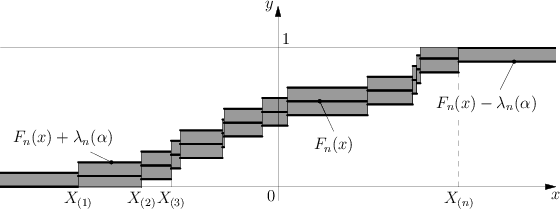# Kolmogorov test

2010 Mathematics Subject Classification: Primary: 62G10 [MSN][ZBL]

A statistical test used for testing a simple non-parametric hypothesis $H _ {0}$, according to which independent identically-distributed random variables $X _ {1} \dots X _ {n}$ have a given distribution function $F$, where the alternative hypothesis $H _ {1}$ is taken to be two-sided:

$$| {\mathsf E} F _ {n} ( x) - F ( x) | > 0 ,$$

where ${\mathsf E} F _ {n}$ is the mathematical expectation of the empirical distribution function $F _ {n}$. The critical set of the Kolmogorov test is expressed by the inequality

$$D _ {n} = \ \sup _ {| x | < \infty } \ | F _ {n} ( x) - F ( x) | > \lambda _ {n}$$

and is based on the following theorem, proved by A.N. Kolmogorov in 1933: If the hypothesis $H _ {0}$ is true, then the distribution of the statistic $D _ {n}$ does not depend on $F$; also, as $n \rightarrow \infty$,

$${\mathsf P} \{ \sqrt n D _ {n} < \lambda \} \rightarrow K ( \lambda ) ,\ \ \lambda > 0 ,$$

where

$$K ( \lambda ) = \ \sum _ {m = - \infty } ^ \infty ( - 1 ) ^ {m} e ^ {- 2 m ^ {2} \lambda ^ {2} } .$$

In 1948 N.V. Smirnov [BS] tabulated the Kolmogorov distribution function $K ( \lambda )$. According to the Kolmogorov test with significance level $\alpha$, $0 < \alpha < 0.5$, the hypothesis $H _ {0}$ must be rejected if $D _ {n} \geq \lambda _ {n} ( \alpha )$, where $\lambda _ {n} ( \alpha )$ is the critical value of the Kolmogorov test corresponding to the given significance level $\alpha$ and is the root of the equation ${\mathsf P} \{ D _ {n} \geq \lambda \} = \alpha$.

To determine $\lambda _ {n} ( \alpha )$ one recommends the use of the approximation of the limiting law of the Kolmogorov statistic $D _ {n}$ and its limiting distribution; see [B], where it is shown that, as $n \rightarrow \infty$ and $0 < \lambda _ {0} < \lambda = O ( n ^ {1/3} )$,

$$\tag{* } {\mathsf P} \left \{ \frac{1}{18n} ( 6 n D _ {n} + 1 ) ^ {2} \geq \lambda \right \} =$$

$$= \ \left [ 1 - K \left ( \sqrt { \frac \lambda {2} } \right ) \right ] \left [ 1 + O \left ( \frac{1}{n} \right ) \right ] .$$

The application of the approximation (*) gives the following approximation of the critical value:

$$\lambda _ {n} ( \alpha ) \approx \ \sqrt { \frac{z}{2n} } - \frac{1}{6n} ,$$

where $z$ is the root of the equation $1 - K ( \sqrt {z/2 } ) = \alpha$.

In practice, for the calculation of the value of the statistic $D _ {n}$ one uses the fact that

$$D _ {n} = \ \max ( D _ {n} ^ {+} , D _ {n} ^ {-} ) ,$$

where

$$D _ {n} ^ {+} = \ \max _ {1 \leq m \leq n } \ \left ( \frac{m}{n} - F ( X _ {(} m) ) \right ) ,$$

$$D _ {n} ^ {-} = \max _ {1 \leq m \leq n } \left ( F ( X _ {(} m) ) - m- \frac{1}{n} \right ) ,$$

and $X _ {(} 1) \leq \dots \leq X _ {(} n)$ is the variational series (or set of order statistics) constructed from the sample $X _ {1} \dots X _ {n}$. The Kolmogorov test has the following geometric interpretation (see Fig.).The graph of the functions $F _ {n} ( x)$, $F _ {n} ( x) \pm \lambda _ {n} ( \alpha )$ is depicted in the $xy$- plane. The shaded region is the confidence zone at level $1 - \alpha$ for the distribution function $F$, since if the hypothesis $H _ {0}$ is true, then according to Kolmogorov's theorem

$${\mathsf P} \{ F _ {n} ( x) - \lambda _ {n} ( \alpha ) < F ( x) < F _ {n} ( x) + \lambda _ {n} ( \alpha ) \} \approx 1 - \alpha .$$

If the graph of $F$ does not leave the shaded region then, according to the Kolmogorov test, $H _ {0}$ must be accepted with significance level $\alpha$; otherwise $H _ {0}$ is rejected.

The Kolmogorov test gave a strong impetus to the development of mathematical statistics, being the start of much research on new methods of statistical analysis lying at the foundations of non-parametric statistics.

How to Cite This Entry:
Kolmogorov test. Encyclopedia of Mathematics. URL: http://encyclopediaofmath.org/index.php?title=Kolmogorov_test&oldid=47517
This article was adapted from an original article by M.S. Nikulin (originator), which appeared in Encyclopedia of Mathematics - ISBN 1402006098. See original article